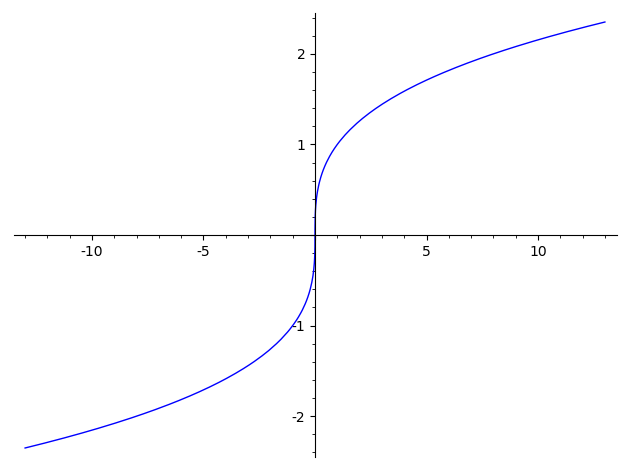# Revision history [back]

SageMath chooses complex cube roots by default, which explains this behavior.

plot(sgn(x)*abs(x)^(1/3),(x,-13,13))SageMath sometimes chooses complex cube roots by default, roots, which explains this behavior.

plot(sgn(x)*abs(x)^(1/3),(x,-13,13))